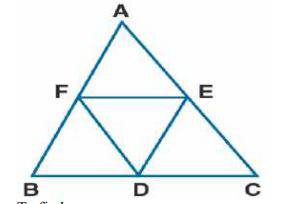# ML Aggarwal Solutions Class 9 Mathematics Solutions for Mid Point Theorem Exercise 11 in Chapter 11 - Mid Point Theorem

If D, E and F are mid-points of sides AB, BC and CA respectively of an isosceles triangle ABC, prove

that Δ DEF is also isosceles.

It is given that

ABC is an isosceles triangle in which AB = AC

D, E, and F are the midpoints of the sides BC, CA, and AB

Now D, E, and F are joinedTo find:

Δ DEF is an isosceles triangle

Proof:

D and E are the midpoints of BC and AC

Here DE || AB and DE = ½ AB ….. (1)

D and F are the midpoints of BC and AB

Here DF || AC and DF = ½ AC ….. (2)

It is given that

AB = BC and DE = DF

Hence, Δ DEF is an isosceles triangle.

Related Questions
Exercises

Lido

Courses

Teachers

Book a Demo with us

Syllabus

Maths
CBSE
Maths
ICSE
Science
CBSE

Science
ICSE
English
CBSE
English
ICSE
Coding

Terms & Policies

Selina Question Bank

Maths
Physics
Biology

Allied Question Bank

Chemistry
Connect with us on social media!